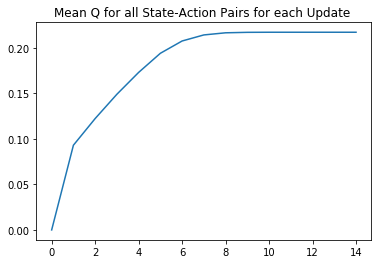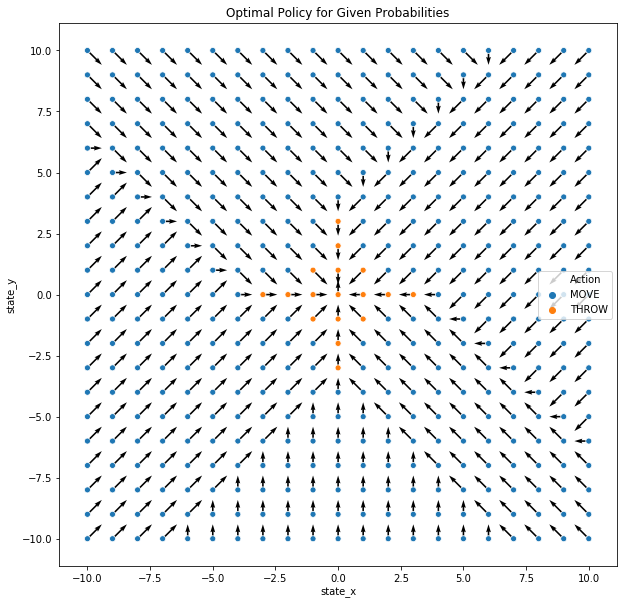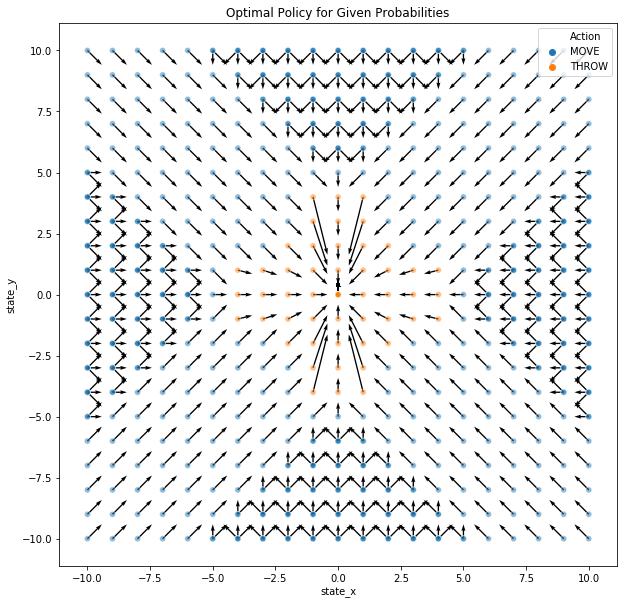# RL from Scratch Part 1.3: Finding the Optimal Policy 2

RL from scratch v1.3

# Reinforcement Learning from Scratch Part 1: Finding the Optimal Policy of an Environment Fully Defined within a Python Notebook¶

## Solving an Example Task of Throwing Paper into a Bin¶

This notebook attempts to solve a basic task of throwing paper into a bin using reinforcement learning. In this problem, we may throw from any position in the room but the probability of it is relative to the current distance from the bin and the direction in which the paper is thrown. Therefore the actions available are to throw the paper in any 360 degree direction or move to a new position to try and increase the probability that a throw made will go into the bin.

We first introduce the problem where the bin’s location is known and can be solved directly with Value-Itearation methods before showing how RL can be used similarly to find the optimal policy if the probabilities are hidden. Furthermore, we introduce the option to add control to the environment where, for example, we can punish the algorithm less for missed throws so that the algorithm will take higher risks.

Lastly, we demonstrate how the envrionment can be changed and, for example, may have walls blocking throws from certain positions.

### 2.4 Increasing the number of iterations¶

We now re-apply the value-iteration algorithm and the subsequent evaluation but increase the number of repeats from 5 to 10 and find that our policy coverges to the optimal one within this.

##### To demonstrate the change over each iteration further, I have included an export of the quiver plot analysis for each iteration and combined these into a small animation. The export is commented out in this published notebook but can be used in your own.¶
In :
input_table = Q_table_VI.copy()
gamma = 0.8
num_repeats = 15

start_time = time.time()

output_metric_table = pd.DataFrame()
# Repeat until converges
for repeats in range(0,num_repeats):

.... OMMITED FOR COMPACTNESS (SAME AS PREVIOUS CODE CELLS) ....

end_time = time.time()

print("total time taken this loop: ", np.round((end_time - start_time)/60,2), " minutes")

Repeats completed:  100.0 %
total time taken this loop:  35.68  minutes

In :
plt.plot(range(0,len(output_metric_table)), output_metric_table['mean_V'])
plt.title("Mean Q for all State-Action Pairs for each Update ")
plt.show()In :
# Create Quiver plot showing current optimal policy in one cell
arrow_scale = 0.1

.... OMMITED FOR COMPACTNESS (SAME AS PREVIOUS CODE CELLS) ....In :
optimal_action_list [ (optimal_action_list['state_x']==-10) & (optimal_action_list['state_y']==0)].head()

Out:
state_x state_y move_dir throw_dir Action move_x move_y throw_dir_2 u v
10 -10 0 1 none MOVE 1 1 -1000 0.1 0.1
##### This figure shows the optimal policy defining the best action at each state.¶

This may seem unusual at first as, for example, state (-10,0) seems as though it should move directly east if it wants to reach the goal faster but if we count the number of steps, as shown below, this is equal.

In fact, there are a number of optimal actions for many of the move states. Any state nots in the diagonal streams can move in any direction that tends towards to bin and still be optimal. i.e. state (-10,0) can move north-east, east or south-east and still be an optimal action. We have currently simply chosen the first one that appears but if we correct this we have the complete optimal policy shown below.

Furthermore, there may be some states where moving is just as optimal as throwing and we therefore reduce the alpha of each point’s colour in an attempt to visualise those that have both.

In :
Q_table_VI_3 [ (Q_table_VI_3['state_x']==-5) & (Q_table_VI_3['state_y']==-5)].sort_values('Q', ascending=False).head()

Out:
throw_dir move_dir state_x state_y Q reward V prob
159547 none 1 -5 -5 0.262144 0 0.262144 1.0
159546 none 0 -5 -5 0.225071 0 0.262144 1.0
159548 none 2 -5 -5 0.225071 0 0.262144 1.0
159553 none 7 -5 -5 0.187998 0 0.262144 1.0
159549 none 3 -5 -5 0.187998 0 0.262144 1.0

#### Save Optimal Policy for Later Comparison¶

This final policy is the one we will consider optimal

In :
# SAVE OPTIMAL POLICY FOR LATER COMPARISON
Optimal_Policy_VI = optimal_action_list.copy()
Optimal_Policy_VI.to_csv('E:\Documents\RL\RL from scratch v2\OptimalPolicy_angletol=45.csv')


#### Change reward for each step to be equal to a small negative value. i.e. want to reach goal faster¶

Aside from changing the two previously mentioned parameters, we can also introduce rewards for each state-action pair. We could use this to define some bias in favour or against specific actions or could more generally decide we want the optimal policy to be more risky and increase the value of throwing.

If, for example, we set the reward of each action equal to a small negative value, our calculations will value throwing slightly less than before but will consider moving even worse in some states. Therefore, more states will be incetivsed to throw instead of move.

In :
input_table = Q_table_VI.copy()
input_table['reward'] = -0.05
gamma = 0.8
num_repeats = 10

start_time = time.time()

output_metric_table = pd.DataFrame()
# Repeat until converges
for repeats in range(0,num_repeats):
clear_output(wait=True)
state_sub_full = pd.DataFrame()

.... OMMITED FOR COMPACTNESS (SAME AS PREVIOUS CODE CELLS) ....

input_table = state_sub_full.copy()
print("Repeats completed: ", np.round((repeats+1)/num_repeats,2)*100, "%")

end_time = time.time()

print("total time taken this loop: ", np.round((end_time - start_time)/60,2), " minutes")

Repeats completed:  100.0 %
total time taken this loop:  25.09  minutes

In :
# Create Quiver plot showing current optimal policy in one cell
arrow_scale = 0.1

Q_table_VI_3 = state_sub_full.copy()

optimal_action_list = pd.DataFrame()
for x in range(0,21):
state_x = int(-10 + x)
for y in range(0,21):
state_y = int(-10 + y)

Q_table_VI_3

for i in range(0,len(Q_table_VI_3[ (Q_table_VI_3['state_x']==state_x) & (Q_table_VI_3['state_y']==state_y) &  (Q_table_VI_3['Q'] == max(Q_table_VI_3[(Q_table_VI_3['state_x']==state_x) &
(Q_table_VI_3['state_y']==state_y)]['Q']))].reset_index(drop=True)['move_dir'])):
optimal_action = pd.DataFrame({'state_x':state_x, 'state_y': state_y,
'move_dir': Q_table_VI_3[ (Q_table_VI_3['state_x']==state_x) & (Q_table_VI_3['state_y']==state_y) &  (Q_table_VI_3['Q'] == max(Q_table_VI_3[(Q_table_VI_3['state_x']==state_x) &
(Q_table_VI_3['state_y']==state_y)]['Q']))].reset_index(drop=True)['move_dir'][i],
'throw_dir': Q_table_VI_3[ (Q_table_VI_3['state_x']==state_x) & (Q_table_VI_3['state_y']==state_y) &  (Q_table_VI_3['Q'] == max(Q_table_VI_3[(Q_table_VI_3['state_x']==state_x) &
(Q_table_VI_3['state_y']==state_y)]['Q']))].reset_index(drop=True)['throw_dir']},
index = [state_y])
optimal_action_list = optimal_action_list.append(optimal_action)
optimal_action_list = optimal_action_list.reset_index(drop=True)

optimal_action_list['Action'] = np.where( optimal_action_list['move_dir'] == 'none', 'THROW', 'MOVE'  )

optimal_action_list['move_x'] = np.where(optimal_action_list['move_dir'] == 0, int(0),
np.where(optimal_action_list['move_dir'] == 1, int(1),
np.where(optimal_action_list['move_dir'] == 2, int(1),
np.where(optimal_action_list['move_dir'] == 3, int(1),
np.where(optimal_action_list['move_dir'] == 4, int(0),
np.where(optimal_action_list['move_dir'] == 5, int(-1),
np.where(optimal_action_list['move_dir'] == 6, int(-1),
np.where(optimal_action_list['move_dir'] == 7, int(-1),
int(-1000)
))))))))
optimal_action_list['move_y'] = np.where(optimal_action_list['move_dir'] == 0, int(1),
np.where(optimal_action_list['move_dir'] == 1, int(1),
np.where(optimal_action_list['move_dir'] == 2, int(0),
np.where(optimal_action_list['move_dir'] == 3, int(-1),
np.where(optimal_action_list['move_dir'] == 4, int(-1),
np.where(optimal_action_list['move_dir'] == 5, int(-1),
np.where(optimal_action_list['move_dir'] == 6, int(0),
np.where(optimal_action_list['move_dir'] == 7, int(1),
int(-1000)
))))))))
optimal_action_list['throw_dir_2'] = np.where(optimal_action_list['throw_dir']=="none",int(-1000), optimal_action_list['throw_dir'])

# Define horizontal arrow component as 0.1*move direction or 0.1/-0.1 depending on throw direction
optimal_action_list['u'] = np.where(optimal_action_list['Action']=="MOVE", optimal_action_list['move_x']*arrow_scale,
np.where(optimal_action_list['throw_dir_2']==0, 0,np.where(optimal_action_list['throw_dir_2']==180, 0,
np.where(optimal_action_list['throw_dir_2']==90, arrow_scale ,np.where(optimal_action_list['throw_dir_2']==270, -arrow_scale,
np.where(optimal_action_list['throw_dir_2']<180, arrow_scale,-arrow_scale))))))

# Define vertical arrow component based 0.1*move direciton or +/- u*tan(throw_dir) accordingly
optimal_action_list['v'] = np.where(optimal_action_list['Action']=="MOVE", optimal_action_list['move_y']*arrow_scale,
np.where(optimal_action_list['throw_dir_2']==0, arrow_scale,np.where(optimal_action_list['throw_dir_2']==180, -arrow_scale,
np.where(optimal_action_list['throw_dir_2']==90, 0,np.where(optimal_action_list['throw_dir_2']==270, 0,

x = optimal_action_list['state_x']
y = optimal_action_list['state_y']
u = optimal_action_list['u'].values
v = optimal_action_list['v'].values
plt.figure(figsize=(10, 10))
plt.quiver(x,y,u,v,scale=0.5,scale_units='inches')
sns.scatterplot( x="state_x", y="state_y", data=optimal_action_list,  hue='Action', alpha = 0.5)
plt.title("Optimal Policy for Given Probabilities")
plt.show()## Conclusion¶

We have defined the environment completely within Python and have the optimal policy calculated using value-iteration. The next step is to try and find the optimal policy using RL given we don’t actually have the probabilities for each state-action pair.

In the next notebook, I will show how this can be computed using Q-learning or Monte Carlo methods.